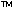DOC HOME SITE MAP MAN PAGES GNU INFO SEARCH PRINT BOOK
Floating point operations

# Exceptions, sticky bits, and trap bits

Floating point operations can lead to any of the following types of floating point exceptions:

• Divide by zero exception

This exception happens when a non-zero number is divided by floating point zero.

• Invalid operation exception

All operations on signaling NaNs raise an invalid operation exception. Zero divided by zero, infinity subtracted from infinity, infinity divided by infinity all raise this exception. When a quiet NaN is compared with the greater or lesser relational operators, an invalid operation exception is raised.

• Overflow exception

This exception occurs when the result of any floating point operation is too large in magnitude to fit in the intended destination.

• Underflow exception

When the underflow trap is enabled, an underflow exception is signaled when the result of some operation is a very tiny non-zero number that may cause some other exception later (such as overflow upon division). When the underflow trap is disabled, an underflow exception occurs only when both the result is very tiny (as explained above) and a loss of accuracy is detected.

• Inexact or imprecise exception

This exception is signaled if the rounded result of an operation is not identical to the infinitely precise result. Inexact exceptions are quite common. 1.0 / 3.0 is an inexact operation. Inexact exceptions also occur when the operation overflows without an overflow trap.

NOTE: The above examples for the exception types do not constitute an exhaustive list of the conditions when an exception can occur.

The floating point implementation on the Intelprocessors includes another exception type called ``Denormalization exception''. This exception occurs when the result of an expression is a denormalized number.

Next topic: Single-precision floating point operations
Previous topic: Rounding control